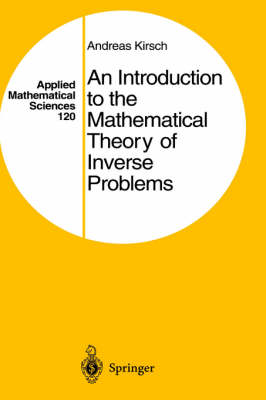•# An Introduction to the Mathematical Theory of Inverse Problems - Applied Mathematical Sciences v. 120 (Hardback)

(author)
£82.50
Hardback 310 Pages / Published: 26/09/1996
• Not available

This product is currently unavailable.

This book introduces the reader to the area of inverse problems. A relatively new branch of Applied Mathematics, the study of inverse problems is of vital interest to many areas of science and technology such as geophysical exploration, system identification, nondestructive testing and ultrasonic tomography. The aim of this book is twofold: in the first part, the reader is exposed to the basic notions and difficulties encountered with ill-posed problems. Basic properties of regularization methods for linear ill-posed problems are studied by means of several simple analytical and numerical examples.The second part of the book presents two special nonlinear inverse problems in detail - the inverse spectral problem and the inverse scattering problem. The corresponding direct problems are studied with respect to existence, uniqueness and continuous dependence on parameters. Then some theoretical results as well as numerical procedures for the inverse problems are discussed. The choice of material and its presentation in the book are new, thus making it particularly suitable for graduate students. Basic knowledge of real analysis is assumed.

Publisher: Springer-Verlag New York Inc.
ISBN: 9780387945309
Number of pages: 310
Weight: 598 g
Dimensions: 235 x 155 x 19 mm